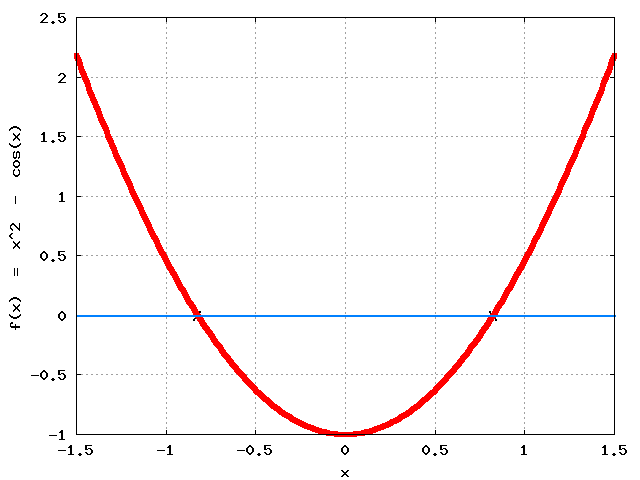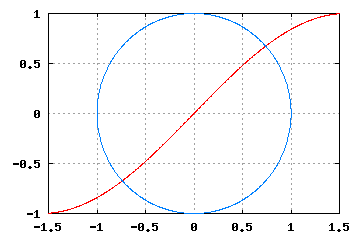Solving one nonlinear algebraic equation
To numerically solve the equation    x^2 - cos(x) = 0,   type
```  Variable  x
Solve( x^2 - cos(x) = 0,   x = 0.2 )   % x = 0.2 is a guess.
```

As graphed to the right, this nonlinear equation has two solutions.
In general, nonlinear equations have an unknown number of solutions (0, 1, 2, 37, ...).
The   Solve   command frequently converges to a solution close to the starting guess.
 If you guess x = 0.2, Solve   reports x = 0.8241323 If you guess x = -9, Solve   reports x = -0.8241323

To save input (for subsequent re-use) and/or input and output responses, type
Save   SolveSampleNonlinearEqn.txt
Save   SolveSampleNonlinearEqn.htmlSolving sets of nonlinear algebraic equations Equation for a circle: x2   +   y2   =   R2 Equation for a sine curve: y   =   A * sin(x) To numerically solve the previous set of nonlinear equations for x and y, type Note: The arguments   x = 3   and   y = 5   are a guess for the solution.
```  Constant  R = 1 meter,  A = 1 meter
Variable  x, y
eqns = x^2 +  y^2  -  R^2       % Circle
eqns = y - A*sin(x)             % Sine wave
Solve( eqns = 0,   x = 3, y = 5 )
```These nonlinear equations can converge to two solutions (depending on the guess).
 If you guess x = 3, y = 5, -> x = 0.739085, y = 0.673612 If you guess x = -2, y = -2, -> x = -0.739085, y = -0.673612

To save input (for subsequent re-use) and/or input and output responses, type
Save   SolveSampleNonlinearEqns.txt
Save   SolveSampleNonlinearEqns.html

 Optional: Use the Input command to vary R or A. Input R := 1.3 m, A := 0.4 m Solve(eqns = 0, x = 3, y = 5) Result: x = 1.243594 Result: y = 0.378778

Advanced: Coding sets of nonlinear algebraic equations
 Equation for a circle: x2   +   y2   =   R2 Equation for a sine curve: y   =   A * sin(x)

 To write a MATLAB® code to numerically solve for x and y for   1 <= R <= 3,   type Constant R, A = 1 meter Variable x, y eqns = x^2 + y^2 - R^2 % Circle eqns = y - A*sin(x) % Sine wave Input x = 3 meters, y = 3 meters Output t seconds, x meters, y meters Code Nonlinear(eqns = 0, x, y) [R = 1, 3, 0.5] SolveSampleNonlinearEqns.m ``` % FILE: SolveSampleNonlinearEqns.1 % % ResidualSum R x y % (UNITS) (meters) (meters) (meters) 1.221245E-15 1.000000E+00 7.390851E-01 6.736120E-01 1.176836E-14 1.500000E+00 1.180882E+00 9.249417E-01 3.482770E-13 2.000000E+00 1.740241E+00 9.856786E-01 2.208789E-13 2.500000E+00 2.408850E+00 6.689109E-01 4.655998E-15 3.000000E+00 2.996515E+00 1.445697E-01 ```MotionGenesis produces highly efficient and symbolically optimized codes.
This short   script   shows how to generate various codes to solve the previous nonlinear equations.